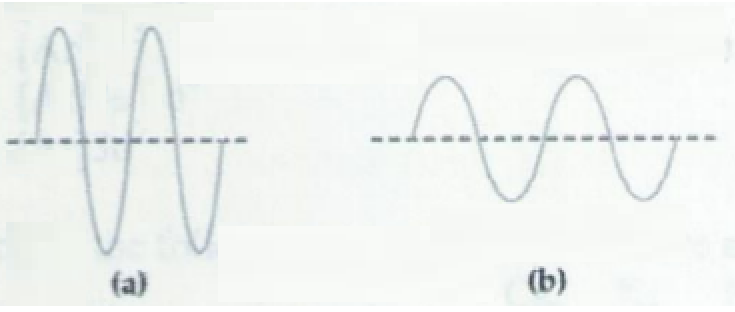# Problem: Two electromagnetic waves are represented below.Wave (b) has thea) higher frequency and higher energy than Wave (a).b) lower frequency and lower energy than wave (a)c) higher frequency and lower energy than wave (a).d) lower frequency and higher energy than wave (a).e) more information is needed.

###### FREE Expert Solution
91% (353 ratings)
###### Problem Details

Two electromagnetic waves are represented below.

Wave (b) has the

a) higher frequency and higher energy than Wave (a).

b) lower frequency and lower energy than wave (a)

c) higher frequency and lower energy than wave (a).

d) lower frequency and higher energy than wave (a).What scientific concept do you need to know in order to solve this problem?

Our tutors have indicated that to solve this problem you will need to apply the Electromagnetic Spectrum concept. If you need more Electromagnetic Spectrum practice, you can also practice Electromagnetic Spectrum practice problems.

What is the difficulty of this problem?

Our tutors rated the difficulty ofTwo electromagnetic waves are represented below.Wave (b) has...as medium difficulty.

How long does this problem take to solve?

Our expert Chemistry tutor, Sabrina took 3 minutes and 11 seconds to solve this problem. You can follow their steps in the video explanation above.

What professor is this problem relevant for?

Based on our data, we think this problem is relevant for Professor Huffman's class at WMU.